Part 1

1. 假設台灣大學資訊系在2001、2002及2003年的人員組成如下表所示：

 類別 大一 大二 大三 大四 碩一 碩二 博士班 教職員 年份 2001 10 21 23 14 35 26 47 48 2002 21 32 33 24 35 26 17 48 2003 15 23 23 44 25 34 27 38

1. 請用 bar3 指令來畫出上述資料的立體長條圖。
2. 請用各種你可以想到的方法，在上述圖形中加入各種解釋和說明的文字。
2. 請使用上題的資料來進行下列兩小題：
1. 畫出依每年份總人數來切分的立體扇形圖，並加上各種可能的說明文字。
2. 重覆上小題，但改成依每種類別的總人數來切分。
3. 畫出下列方程式的曲面圖及等高線圖： $$z=sin(x/2)cos(y)$$ 其中，x 的 21 個值均勻分佈在 [-2*pi, 2*pi] 範圍，y 的 31 值均勻分佈在 [-1.5*pi, 1.5*pi]。請使用 subplot(1,2,1) 和 subplot(1,2,2) 將產生的曲面圖和等高線圖秀在同一個視窗上，產生的圖形應類似下圖：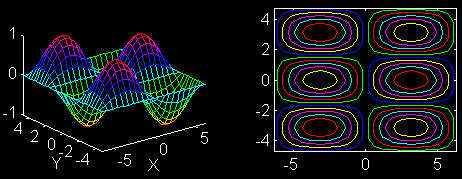4. 請用 meshc 指令來同時畫出下列函數的曲面圖和等高線圖： $$z=\frac{xy}{x+y}$$ 其中 x 和 y 都介於 0 和 1 中間，且各自都分成 21 個格子點，所以此曲面共有 21*21=441 個點。此外，等高線圖應有 20 條。請問此曲面在 XY 平面的哪一點（或區域）會有最大值？此最大值為何？
5. 請畫出下列圓椎曲面的三度空間曲面圖及其10條等高線：
1. $z=xy$
2. $z=x^2+2xy+y^2$
3. $z=x^2+2xy+2y^2$
4. $z=x^2-2xy-y^2$
上述各方程式的定義域皆是 [-10, 10] x [-10, 10]。

Hint
使用 surfc 指令。

6. 假設有一圓球半徑為1，圓心在 (0,0,0)，試畫出此圓球的上半部網狀圖，並將其30條等高線同時畫在曲面的下方。

Hint
使用 meshc 指令。

7. 使用 contour 指令畫出下列隱函數的圖形： $$x^2+y^2=25$$

Hint
畫出z = x2 + y2 在高度等於 25 的一條等高線。

8. 請寫一段程式碼來完成下列繪圖（在進行下列兩項作業時，請顯示複數平面座標）：
1. 參考本章的範例，畫出 $f(z) = |z^4-1|$ 在極座標上的等高線圖。
2. 請同時畫出上述函數的曲面圖和等高線圖。

Part 2

1. Theorem on gradients and contour curves: Consider any point (x0,y0), and the contour curve of f through this point (i.e., the contour curve of f at value f(x0,y0)). Prove that the gradient of f at f(x0,y0) is perpendicular to the tangent direction along the contour curve of f though (x0,y0).
2. Parameter for constructing a crown: Suppose that we have a MATLAB script to generate the crown: theta = -pi:0.05:pi; x = cos(theta); y = sin(theta); z = abs(cos(n*theta)).*exp(-abs(theta/4)); stem3(x, y, z, 'fill'); What is the most likely value of n in order to generate the following crown?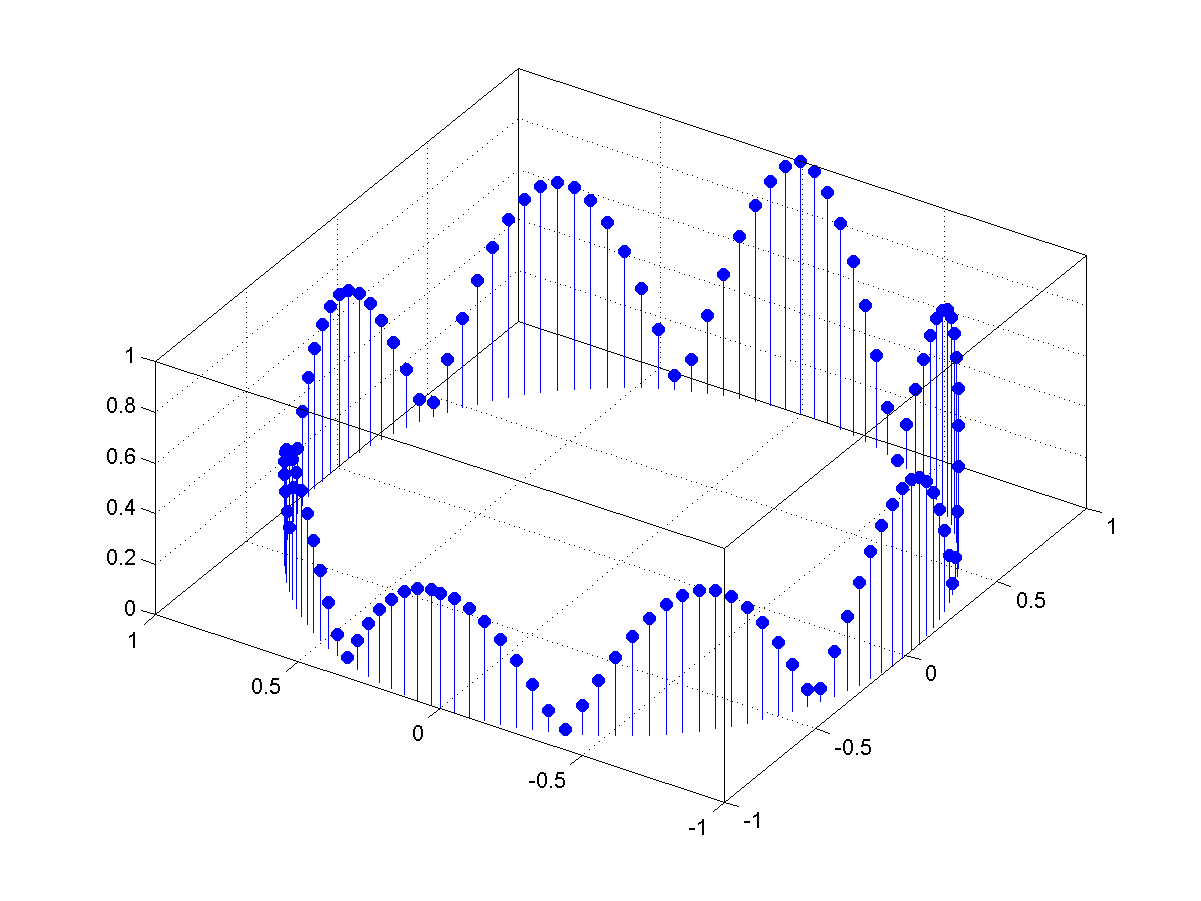3. Construct a crown: Write a MATLAB script to construct a crown as close as possible to the following plot: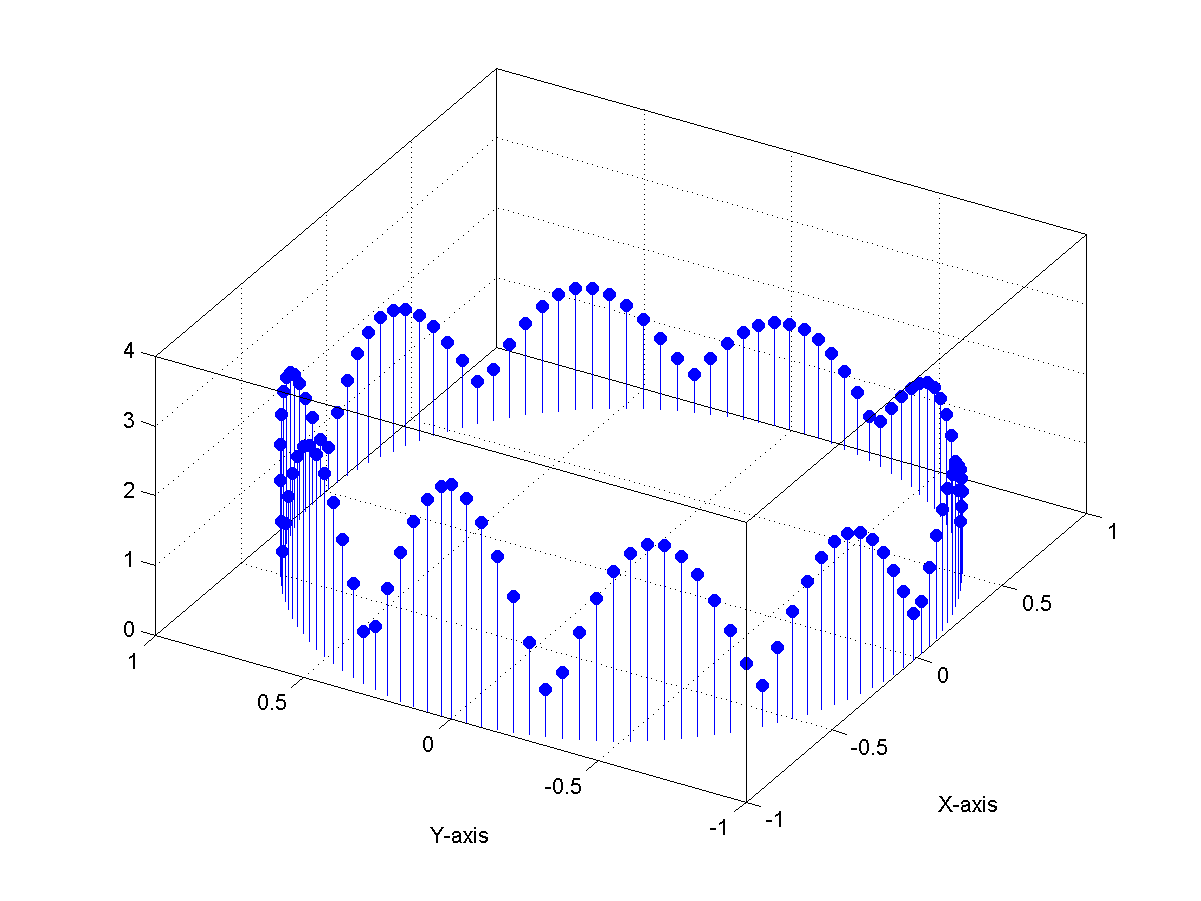4. Construct yet another crown:
1. Plot the function sin(x)/x over the interval [-pi, pi].
2. Plot the function of |sin(x)/x| over the interval [-pi, pi].
3. Write a MATLAB script to construct a crown as close as possible to the following plot: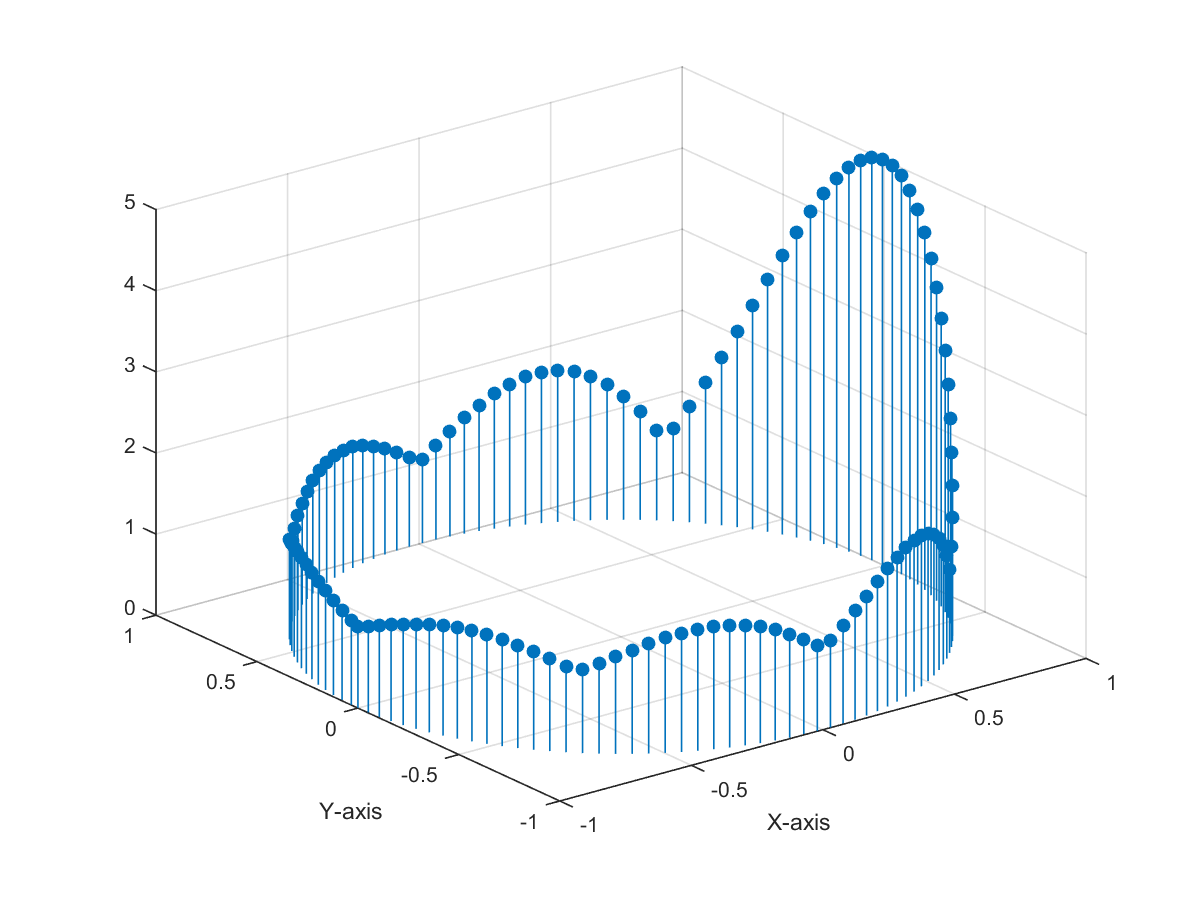5. Interpolation of indexed color: Suppose that we have a MATLAB script to genrate a rectangle of varying color: X = [0 0 1 1]; Y = [0 1 1 0]; Z = [0 1 1 0]; C = [0 0.3 0.6 0.9]; fill3(X, Y, Z, C); colorbar;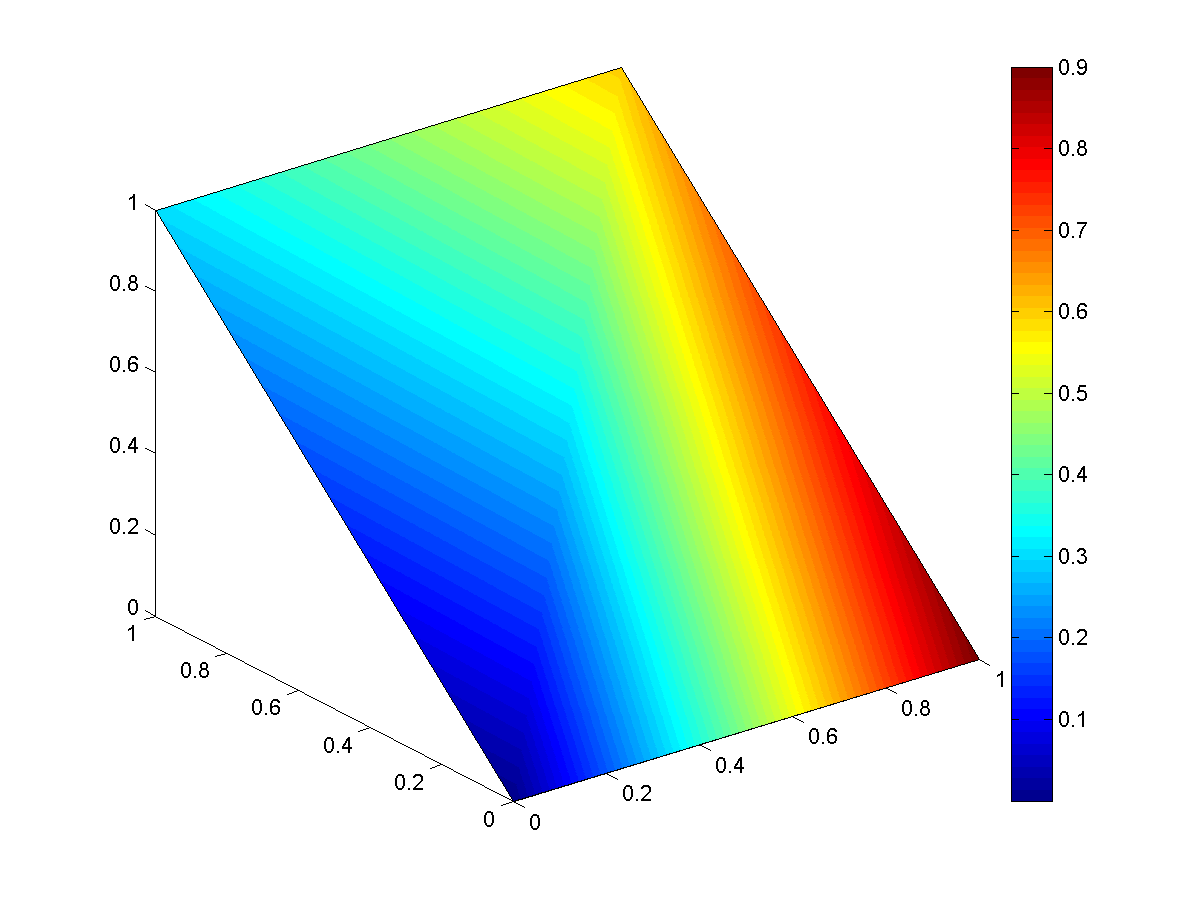What is the color value (in terms of the colormap) at x=2/3 and y=1/2?
6. Scatter data plot and its contours: 請跟據課本範例4─12的曲面，來同時畫出曲面圖及等高線圖：
• 請畫出此曲面的等高線圖。
• 請在等高線圖上面畫出每一個資料點。
• 請疊加畫出這些資料點的 convex hull。（提示：請參考指令 convhull。）
典型結果如下：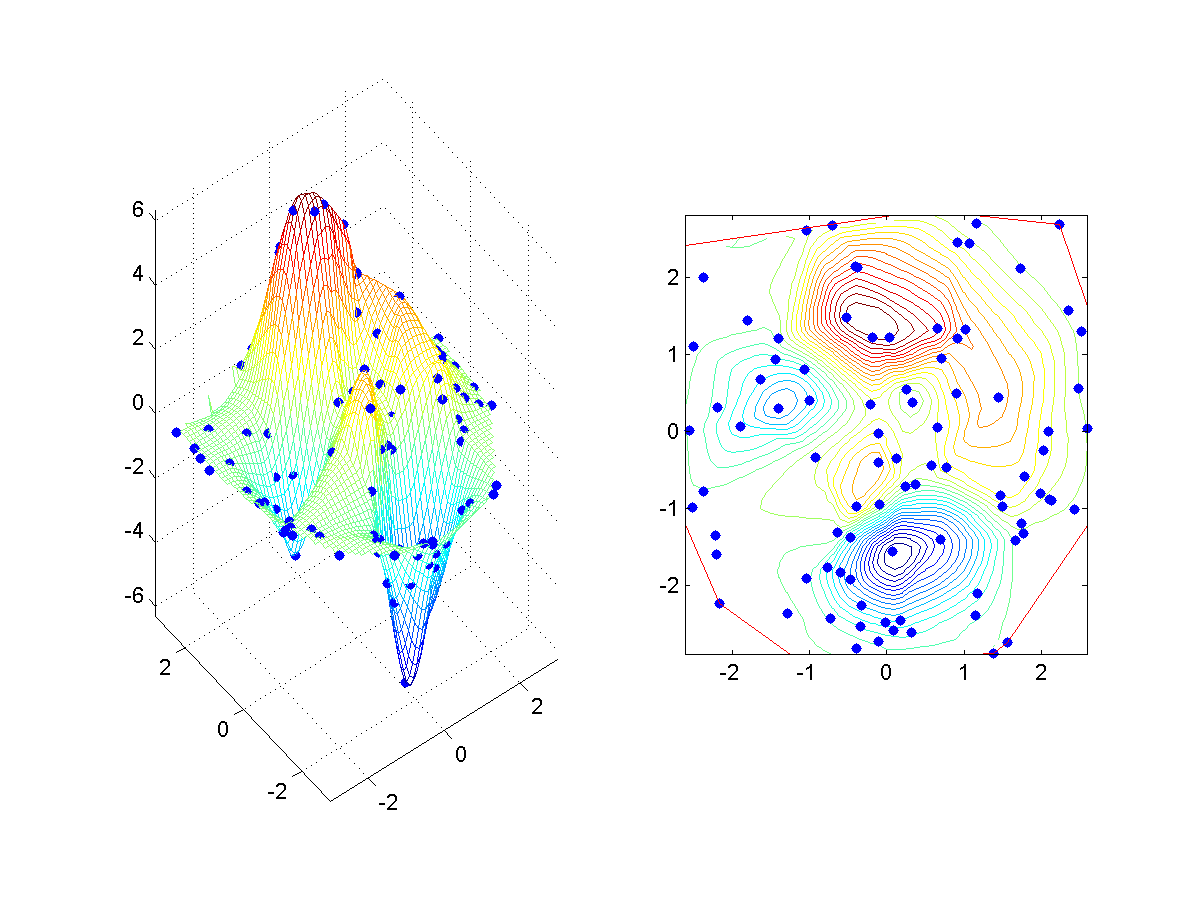7. Multiple plots of peaks function: 請跟據 peaks 來進行下列作圖：
• 請畫出此曲面，曲面矩陣維度是 40x40。
• 加入三度空間的 30 條等高線。
• 再加入三度空間的 surface norm vectors。
典型結果如下：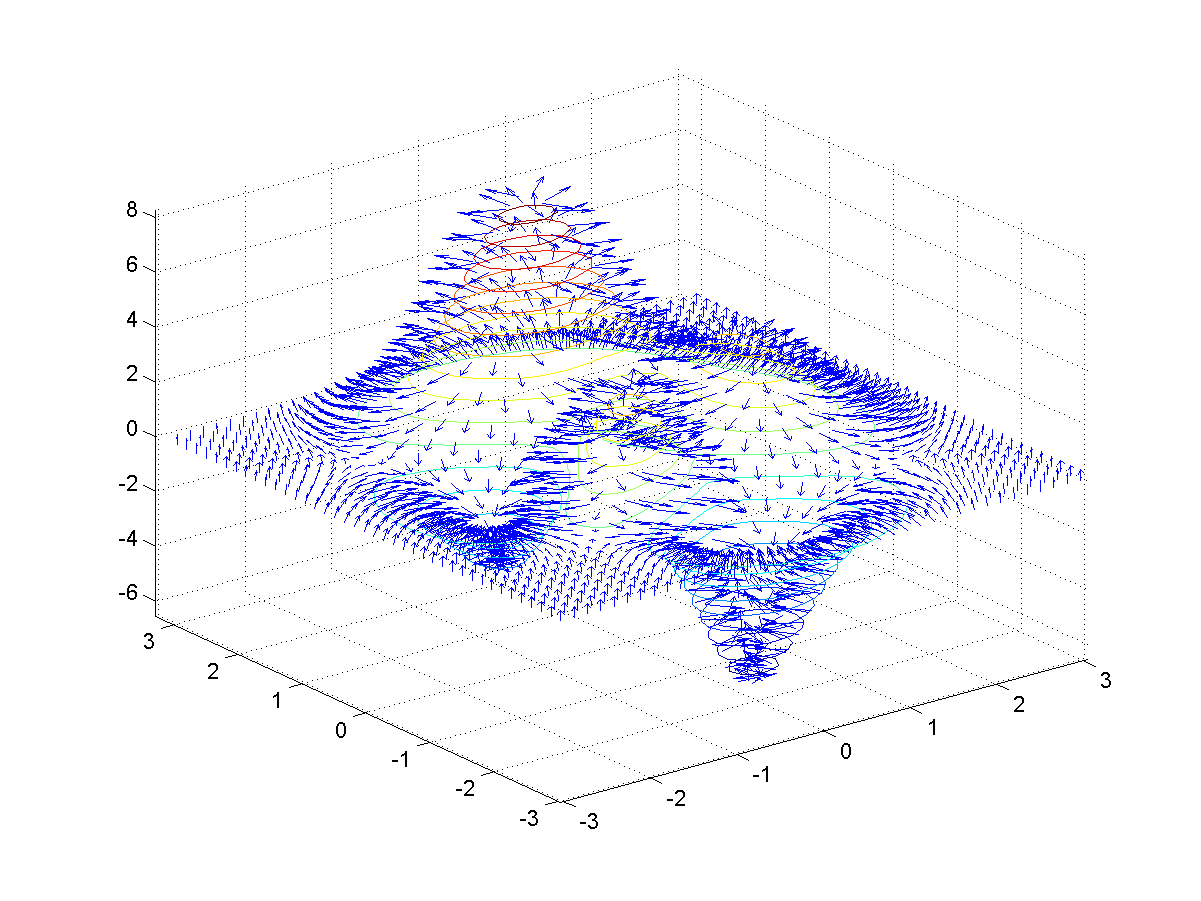MATLAB程式設計：入門篇